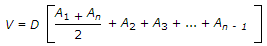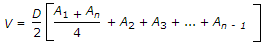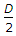# Civil Engineering - Surveying

### Exercise :: Surveying - Section 8

6.

Permanent adjustments of a level are

 A. 2 in number B. 3 in number C. 4 in number D. 6 in number

Explanation:

No answer description available for this question. Let us discuss.

7.

The radius of a simple circular curve is 300 m and length of its specified chord is 30 m. The degree of the curve is

 A. 5.73° B. 5.37° C. 3.57° D. 3.75°.

Explanation:

No answer description available for this question. Let us discuss.

8.

During levelling if back sight is more than foresight

 A. The forward staff is at lower point B. The back staff is at lower point C. The difference in level, cannot be ascertained. D. none of these.

Explanation:

No answer description available for this question. Let us discuss.

9.

Two contour lines, having the same elevation

 A. cannot cross each other B. can cross each other C. cannot unite together D. can unite together.

Explanation:

No answer description available for this question. Let us discuss.

10.

The Trapezoidal rule of volumes V of an embankment divided into a number of sections equidistant D, is given by

 A.B.C. V =[A1 + An + 2(A2 + A4 + ... + An - 1) + 4(A3 + A5 + ... + An - 2)] D. V =[A1 + An + 4(A2 + A4 + ... + An - 1) + 4(A3 + A5 + ... + An - 2)].